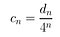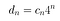# Abstract

Elementary CA Rule 30, when row coefficients are calculated and analysed, appear to express similar characteristics to logistic functions.

Rule 30’s row coefficients appear to resolve to an unstable orbit with a period of 4, hinting at the potential for the existence of a more universal logistic function governing Rule 30’s output.

Logistic functions are used extensively to model population growth. Intuitively, Elementary CA rules can be seen as determining the birth and death rate of a population of cells — some cells cannibalise others, some cells merge together, others create new life as generations progress.

Calculating multiple generations with a logistic function, using the population size of each generation as the coefficient to calculate a “row” of a virtual or simulated “cellular automata” results in some familiar properties, warranting further investigation.

# Background

Elementary Cellular Automata are simple functions which in some cases result in highly complex and unpredictable results.

For example, each row in the illustration below is a new generation, with values for each cell calculated based on a simple comparison with the cell’s neighbour’s in the previous generation, as illustrated below:

Despite the simplicity of the algorithm, the various rules are able to generate everything from simple repeating patterns to pseudo-random chaos. Rule 30 in particular is famous for its apparent non-repeating nature.

Many fundamental questions remain unanswered — while the chaotic nature has been demonstrated through brute force computation and analysis, there is as yet no formal proof tying back into standard number theories that can say one way or another if Rule 30 is always going to be random or if it will start repeating at some point.

Also, it is unknown for sure whether there is a more basic representation or algorithm that provides a “shortcut” to determining the centre value of any generation, without needing to fully compute and store each and every preceding generation. While some optimisations can reduce the overall effort by a certain factor, the algorithm still requires increasing effort as the number of generations increases.

Therefore the study of the relationships and patterns within elementary CA and other mathematical constructs is profoundly interesting. As CA are often used to model physical processes, finding any link to other well established areas could be significantly beneficial.

# Evaluating elementary CA row coefficients

One way of representing a row in elementary CA is to calculate its decimal value.

Since a row is a sequence of active and inactive states, represented here as 1’s and 0’s, a simple binary to decimal conversion gives the decimal value of the row.

Rule 30 when seeded with a single “1” progresses as follows:

• Generation 0: 1 (binary) =1 (decimal)
• Generation 1: 111 (binary) = 7 (decimal)
• Generation 2: 11001 (binary) = 25 (decimal)
• Generation 3: 1101111 (binary) = 111 (decimal)

Each generation’s binary length increases by two places, so the normalised coefficient c for a row’s decimal value d can be calculated as such:Coefficient for row “n” = Decimal value of row “n” divided by 4 to the power of “n”

Thus the coefficients for Rule 30 progress as follows:

• 1
• 1.75
• 1.5625
• 1.73438
• 1.56641

After several generations, the coefficient for rule 30 seems to follow a general pattern.

The cycle does not appear to be periodic, assuming an unstable orbit around 4 values which resemble period doubling bifurcation.

# Visualising Elementary CA Coefficients

I have provided a quick tool to visualise coefficients of elementary CA here.

This tool is fairly rudimentary, and simply generates a number of rows (n) for a given rule, calculating and plotting the coefficient value for each row.

Here’s some of the characteristics of different elementary CA rules.

## Rule 30

50 generations of Rule 30’s coefficients are illustrated below:

Rule 30, despite it’s apparent randomness, has coefficients which appear to quickly resolve around two values, however if we plot 500 generations it’s clearer that the cycle orbits 4 values.

Here again over 5000 generations:

## Rule 101

Rule 101 also exhibits a periodic nature:

Rule 101’s cycle seems a lot more complex, seen here plotted over 5000 generations:

Rule 112

Other rules, such as Rule 112, are not periodic, the coefficient decays quickly towards zero.

# Visualising Logistic Function as CA

If we know ahead of time the coefficient for a particular row n, the decimal value for that row is easily obtained:Decimal value of row “n” = Coefficient of row “n” multiplied by 4 to the power of “n”

The obvious and potentially fatal flaw in this plan, other than the required decimal accuracy to avoid rounding errors, is that there may be no simple function that can easily compute the coefficient of an arbitrary row.

However logistic functions appear at least on a surface level to bear much similarity to the patterns observed in the coefficients of elementary CA.

The standard logistic function below calculates the population size of subsequent generations, given an initial population:

Which can be graphed as follows:

Superimposing population in blue vs the coefficients for rule 30 in red, we can get a close match — although not exact in this scenario — the input rate and initial population could be tweaked endlessly for a closer match — and the magnitude of the value is off somewhat.

Despite values only roughly matching, if we use the generated population values as row coefficients, and graph these in the form of a standard elementary CA, we can see some familiar patterns:

The familiar features include an expanding area of stability / repetition on the left hand side of the chart, and noise / chaos on the right hand side.

What appears to be missing are periodic shapes that recur in the chaotic area, and the regularity of the least significant bit that occurs in Elementary CA Rule 30.

However it is interesting that generating row coefficients from a logistic function appears to come close to resembling the output of rule 30.

Perhaps the defects could be addressed with more care in selecting the correct geometry between initial population and rate, or exploring a variety of resource-limited exponential functions to see if these features can be preserved.

# Other CA-like patterns or similarities:

Various rates and populations provide interesting patterns that echo the pseudo-regular features of many CA, for example with a growth rate of 4:

Or with growth rates ~2.6:

# Implications / Follow-up

Is there a variation or type of logistic function of which Rule 30’s output is a subset?

If there is, then does this imply that Rule 30 (and possibly elementary CA in general) are binary analogues to logistic functions in general?

Could one brute force population and rate values to find a near match in the standard logistic function itself?

I’d probably start by looking somewhere around r=3.2–3.3ish…

If elementary CA can reduce to logistic functions, what are the implications for multi-dimensional CA? Could these be generalised as well?

More investigation seems to be warranted.

# Logistic CA Emulation Tool

The quick tool for generating “fake” CA output with the logistic function is here.

The bit precision of the tools is limited. I have used BigNumber where appropriate however using it is unweildy — so it could well be that we’re just not seeing patterns to the right hand side that should be there — or a lack of patterns that should exist — because the least significant bits are approximated.

The logistic function most probably (I guess) will not magically generate Rule 30, at least not beyond a few initial rows. The interesting bit is the correlation in features, specifically the correlation between row co-efficient values from Rule 30, and population growth dynamics from the logistic function.

# Final thoughts

With the limited real number accuracy in my code, the rounding errors in the least significant bits add up quite quickly when calculating each generation, so I have only been able to view and manipulate approximate patterns.

Using a BigNumber library, I can only calculate ~10 rows in any kind of reasonable time. I could achieve speed gains by using a precompiled binary, however the fact remains that all things equal, calculating the Cellular Automata rows themselves is far quicker than using high-precision decimals once more than a tiny number of rows is required.

The hunch I gather from this exercise is that it seems more likely to me that, if a relationship exists, the cellular automata are simplified subsets of a more universal function or functions, with breadcrumbs scattered toward some kind of logistic function, if they’re linked to anything at all.